# uiaxes

Create UI axes for plots in apps

## Syntax

``ax = uiaxes``
``ax = uiaxes(Name,Value)``
``ax = uiaxes(parent)``
``ax = uiaxes(parent,Name,Value)``

## Description

example

````ax = uiaxes` creates a UI axes in a new figure window and returns the `UIAxes` object. MATLAB® calls the `uifigure` function to create the figure.`UIAxes` objects are useful for creating Cartesian plots in apps. They are very similar to the Cartesian `Axes` objects returned by the `axes` function. Thus, you can pass a `UIAxes` object to most functions that accept an `Axes` object. For more information, see Differences Between UIAxes and Axes Objects.```

example

````ax = uiaxes(Name,Value)` specifies `UIAxes` property values using one or more `Name,Value` pair arguments.```

example

````ax = uiaxes(parent)` creates the UI axes in the specified parent container. The parent can be a `Figure` created using the `uifigure` function, or one of its child containers.```
````ax = uiaxes(parent,Name,Value)` specifies `UIAxes` property values using one or more `Name,Value` arguments.```

## Examples

collapse all

Create a line plot and a scatter plot in UI axes.

Create a figure window with UI axes and assign the `UIAxes` object to the variable `ax`. Add a line plot to the axes by specifying the `UIAxes` object as the first input argument for the `plot` function.

```fig = uifigure; ax = uiaxes(fig); x = linspace(-pi,pi,50); y = 5*sin(x); plot(ax,x,y) ```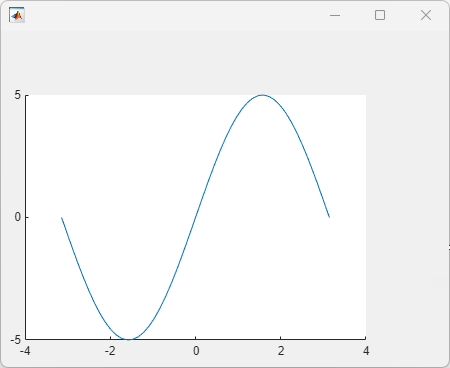Set the hold state on and add a scatter plot. Specify the `UIAxes` object as the first input argument for the `hold` and `scatter` functions.

```hold(ax,'on') y2 = 5*sin(x) + randn(1,50); scatter(ax,x,y2)```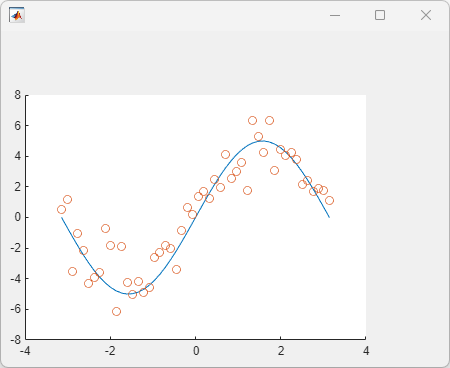Modify the appearance of the UI axes by setting properties using name-value pair arguments. For example, reverse the x-axis direction using the `XDir` name-value pair.

```fig = uifigure; ax = uiaxes(fig,'XDir','reverse'); x = linspace(-pi,pi); y = sin(x); plot(ax,x,y)```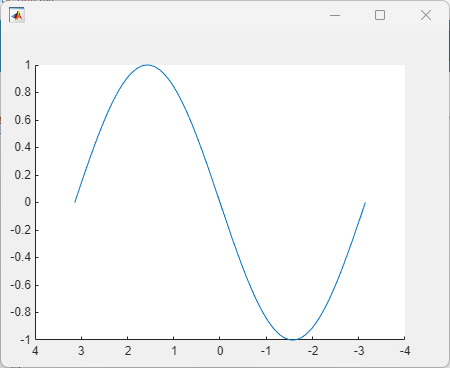Alternatively, specify properties after the axes is created using dot notation. For example, reverse the y-axis direction using dot notation to access the `YDir` property.

`ax.YDir = 'reverse';`

Specify the UI axes position by setting the `Position` property. Specify the position in pixels.

```fig = uifigure; ax = uiaxes(fig,'Position',[10 10 550 400]); ```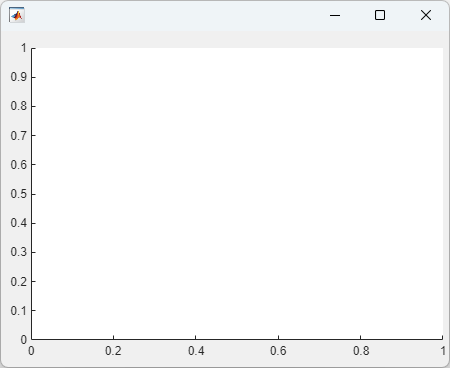Add UI axes to a panel within a figure window. Specify the panel and axes positions in pixels.

```fig = uifigure; p = uipanel(fig,'Position',[10 10 400 400]); ax = uiaxes(p,'Position',[10 10 390 390]);```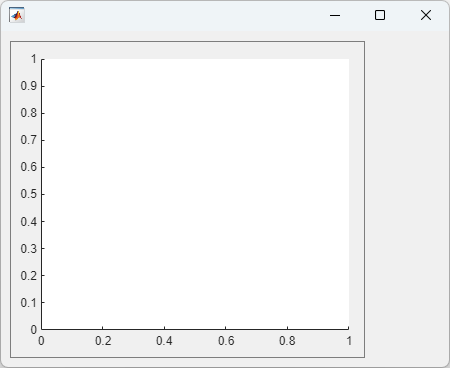## Input Arguments

collapse all

Parent container, specified as a `Figure`, `Panel`, `Tab`, `GridLayout`, or `TiledChartLayout` object. If no container is specified, MATLAB calls the `uifigure` function to create a new `Figure` object that serves as the parent container.

### Name-Value Arguments

Specify optional pairs of arguments as `Name1=Value1,...,NameN=ValueN`, where `Name` is the argument name and `Value` is the corresponding value. Name-value arguments must appear after other arguments, but the order of the pairs does not matter.

Before R2021a, use commas to separate each name and value, and enclose `Name` in quotes.

Example: `'Xscale','linear','YScale','log'`

The properties list here are only a subset. For a full list, see UIAxes Properties.

Minimum and maximum limits, specified as a two-element vector of the form `[min max]`, where `max` is greater than `min`. You can specify the limits as numeric, categorical, datetime, or duration values. However, the type of values that you specify must match the type of values along the axis.

You can specify both limits, or specify one limit and let MATLAB automatically calculate the other. For an automatically calculated minimum or maximum limit, use `-inf` or `inf`, respectively. MATLAB uses the `'tight'` limit method to calculate the corresponding limit.

Example: `ax.XLim = [0 10]`

Example: `ax.YLim = [-inf 10]`

Example: `ax.ZLim = [0 inf]`

Alternatively, use the `xlim`, `ylim`, and `zlim` functions to set the limits. For an example, see Specify Axis Limits.

Data Types: `single` | `double` | `int8` | `int16` | `int32` | `int64` | `uint8` | `uint16` | `uint32` | `uint64` | `datetime` | `duration`

Axis scale, specified as one of these values.

ValueDescriptionResult
`'linear'`

Linear scale

Example: `ax.XScale = 'linear'`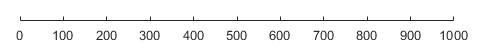`'log'`

Log scale

Example: `ax.XScale = 'log'`

Note

The axes might exclude coordinates in some cases:

• If the coordinates include positive and negative values, only the positive values are displayed.

• If the coordinates are all negative, all of the values are displayed on a log scale with the appropriate sign.

• Zero values are not displayed.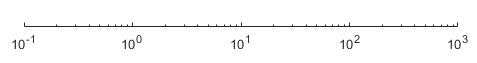Line style for grid lines, specified as one of the line styles in this table.

Line StyleDescriptionResulting Line
`'-'`Solid line`'--'`Dashed line`':'`Dotted line`'-.'`Dash-dotted line`'none'`No lineNo line

To display the grid lines, use the `grid on` command or set the `XGrid`, `YGrid`, or `ZGrid` property to `'on'`.

Example: `ax.GridLineStyle = '--'`

Size and location of axes, including the labels and margins, specified as a four-element vector of the form `[left bottom width height]`. This property is equivalent to the `OuterPosition` property. The vector defines a rectangle that encloses the outer bounds of the axes. The values are measured in the units specified by the `Units` property, which defaults to pixels.

• The `left` and `bottom` elements define the position of the rectangle, measured from the lower left corner of the parent container.

• The `width` and `height` define the size of the rectangle.

If you want to specify the position and account for the text around the axes, then set the either the `Position` or the `OuterPosition` property. These figures show the areas defined by the `Position` (or `OuterPosition`) in blue, and the `InnerPosition` in red.

2-D View of Axes3-D View of Axes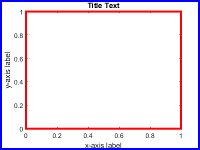Note

Setting this property has no effect when the parent container is a `TiledChartLayout`.

## Output Arguments

collapse all

`UIAxes` object. Use `ax` to set properties of the `UIAxes` after they are created.

collapse all

### Differences Between `UIAxes` and `Axes` Objects

This table describes the properties that are different for `UIAxes` and `Axes` objects. For more information on creating charts in apps, see Display Graphics in App Designer.

Property`UIAxes` Objects`Axes` Objects
`NextPlot`

The default value is `'replacechildren'`.

The default value is `'replace'`.

`Position`

The default `Position` is ```[10 10 400 300]``` in pixels.

The `Position` property is equivalent to the `OuterPosition` property.

The default `Position` is `[0.1300 0.1100 0.7750 0.8150]` in normalized units.

The `Position` property is equivalent to the `InnerPosition` property.

`Units`

The default value is `'pixels'`.

The default value is `'normalized'`.

`FontUnits`

The default value is `'pixels'`.

The default value is `'points'`.

## Version History

Introduced in R2016a